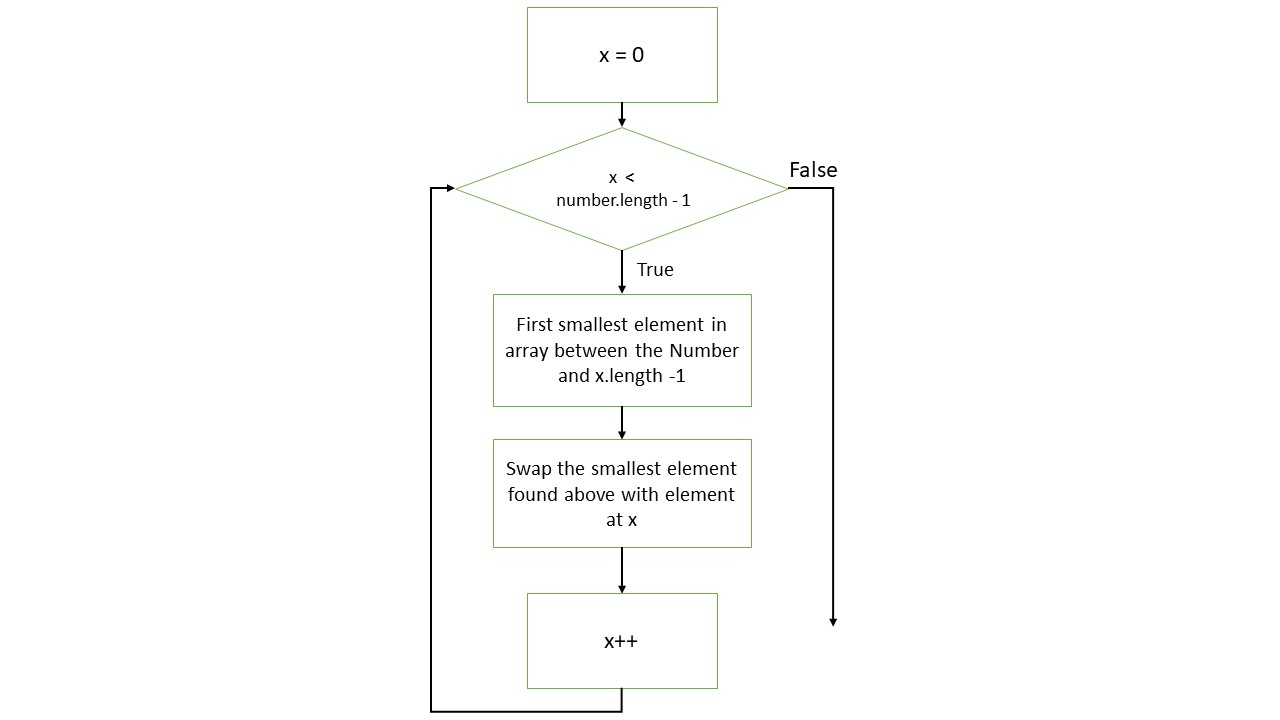# Selection Sort Algorithm in Java

Sheeraz Gul Mar 25, 2022

The selection sort is a method where the smallest element in a list or array is selected first and swapped with the first element or array; then, the second smalled element is swapped with the second element. This process repeats until the entire list or array is sorted.

This tutorial will demonstrate how selection sort works and how to implement it in Java.

## Selection Sort Algorithm in Java

The selection sort algorithm has four main steps:

• ##### Repeat the process till all the elements are swapped to their corresponding positions.

The flowchart below demonstrates the whole process of the selection sort algorithm:## Implement Selection Sort Algorithm in Java

Let’s implement the above algorithm in Java.

Example:

``````package delftstack;

import java.util.*;
public class Selection_Sort {
static void select_sort(int Demo_Array[]) {
int length = Demo_Array.length;

// traversing the unsorted array
for (int x = 0; x < length-1; x++) {
// finding the minimum element in the array
int minimum_index = x;
for (int y = x+1; y < length; y++) {
if (Demo_Array[y] < Demo_Array[minimum_index])
minimum_index = y;
}
// Swapping the elements
int temp = Demo_Array[minimum_index];
Demo_Array[minimum_index] = Demo_Array[x];
Demo_Array[x] = temp;
}
}

public static void main(String args[]){
//Original Unsorted Array
int Demo_Array[] = {6, 2, 1, 45, 23, 19, 63, 5, 43, 50};
System.out.println("The Original Unsorted Array: \n" + Arrays.toString(Demo_Array));
//call selection sort
select_sort(Demo_Array);
//print the sorted array
System.out.println("Sorted Array By the Selection Sort: \n" + Arrays.toString(Demo_Array));
}
}
``````

The code above implements the selection sort on the array as described above.

Output:

``````The Original Unsorted Array:
[6, 2, 1, 45, 23, 19, 63, 5, 43, 50]
Sorted Array By the Selection Sort:
[1, 2, 5, 6, 19, 23, 43, 45, 50, 63]
``````

Let’s try another way by inputting the array size and elements.

Example:

``````package delftstack;

import java.util.Scanner;
import java.util.Arrays;

public class Selection_Sort  {
public static void main(String args[]) {
int Array_Size;
Scanner Array_Scan = new Scanner(System.in);

System.out.print("Enter the size of the Array : ");
Array_Size = Array_Scan.nextInt();
int Demo_Array[] = new int[Array_Size];

System.out.print("Enter the elements of the Array : ");
for(int x=0; x<Array_Size; x++){
Demo_Array[x] = Array_Scan.nextInt();
}

System.out.println("The Original Unsorted Array: \n" + Arrays.toString(Demo_Array));

//Sorting Array using Selection Sort Technique
for(int x=0; x<Array_Size; x++){
for(int y=x+1; y<Array_Size; y++){
if(Demo_Array[x] > Demo_Array[y])  {
int temp = Demo_Array[x];
Demo_Array[x] = Demo_Array[y];
Demo_Array[y] = temp;
}
}
}

System.out.println("The array after selection sort: \n" + Arrays.toString(Demo_Array));
}
}
``````

The code will first ask to input the size of the array and then asks to input the elements of the array and finally sorts the given array using selection sort.

Output:

``````Enter the size of the Array : 10
Enter the elements of the Array :
12
23
45
21
45
64
1
3
45
67
The Original Unsorted Array:
[12, 23, 45, 21, 45, 64, 1, 3, 45, 67]
The array after selection sort:
[1, 3, 12, 21, 23, 45, 45, 45, 64, 67]
``````
Author: Sheeraz Gul

Sheeraz is a Doctorate fellow in Computer Science at Northwestern Polytechnical University, Xian, China. He has 7 years of Software Development experience in AI, Web, Database, and Desktop technologies. He writes tutorials in Java, PHP, Python, GoLang, R, etc., to help beginners learn the field of Computer Science.